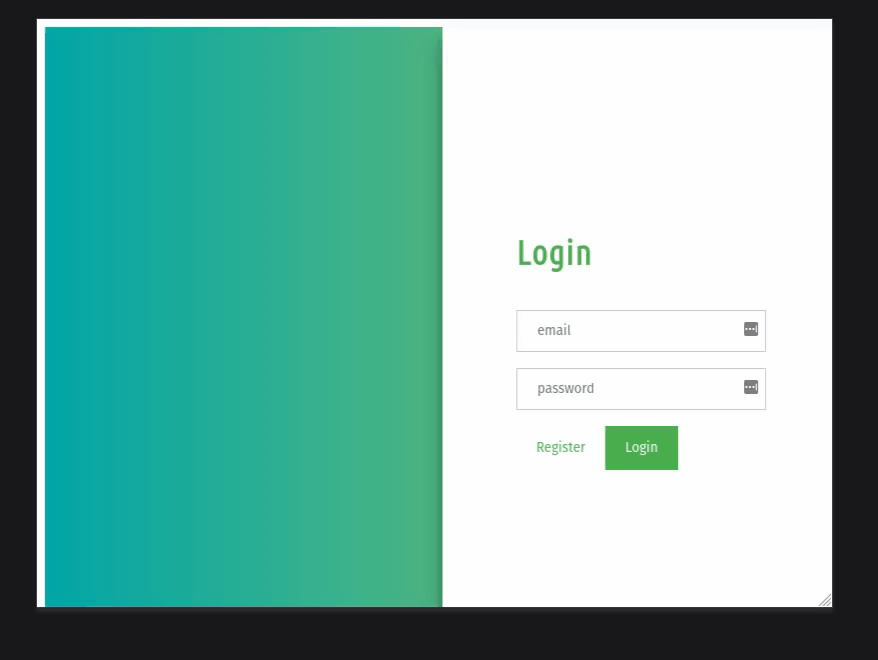# How to make responsive sliding login and registration form using HTML CSS and JavaScript ?

In this article, we will learn how to make responsive sliding login and registration forms using HTML, CSS, and javascript. Forms are used on webpages for the user to enter their required details that further send to the server for processing. A form is also known as a web form or HTML form. In this article, you will learn to make a responsive sliding login and registration form using HTML, CSS, and Javascript

The main crux of this article is to slide form in to-for(left-right) motion when clicking the buttons and this can be done using the animate() method in JQuery which is an inbuilt method in jQuery that is used to change the state of the element with CSS style.

Syntax:

`(selector).animate({styles}, para1, para2, para3);`

Using the animate() method in JQuery is discussed further in this article.

Example: Here is the implementation of the above-explained method.

HTML Code: First we will add HTML code to form the basic structure of the Login and Registration form.

## HTML

 `` `<``html``>` `<``head``>` `    ``<``meta` `name``=``"viewport"` `content``=` `        ``"width=device-width, initial-scale=1"` `/>` `    ``<``script` `src``=` `"https://ajax.googleapis.com/ajax/libs/jquery/3.3.1/jquery.min.js"``>` `    ``` ``   `<``body``>` `    ``<``div` `id``=``"background"``>` `        ``<``div` `class``=``"background-Right"``>` `        ``<``div` `class``=``"background-Left"``>` `    ```   `    ``<``div` `id``=``"slide"``>` `        ``<``div` `class``=``"top"``>` `            ``<``div` `class``=``"left"``>` `                ``<``div` `class``=``"content"``>` `                    ``<``h2``>Sign Up` `                    ``<``form` `method``=``"post"` `                        ``onsubmit``=``"return false;"``>` `                        ``<``div``>` `                            ``<``input` `type``=``"text"` `                                ``placeholder``=``"email"` `/> ` `                            `  `                            ``<``br` `/>` `                            ``<``input` `type``=``"password"` `                                ``placeholder``=``"password"` `/>` `                        ``` `                    ```   `                    ``<``button` `id``=``"LeftToRight"` `                        ``class``=``"on-off"``>Login` `                    ``` `                    `  `                    ``<``button``>Register` `                ``` `            ```   `            ``<``div` `class``=``"right"``>` `                ``<``div` `class``=``"content"``>` `                    ``<``h2``>Login` `                    ``<``form` `method``=``"post"` `                        ``onsubmit``=``"return false;"``>` `                        ``<``div``>` `                            ``<``input` `type``=``"text"` `                                ``placeholder``=``"email"` `/> ` `                            `  `                            ``<``br` `/>` `                            ``<``input` `type``=``"password"` `                                ``placeholder``=``"password"` `/>` `                        ```   `                        ``<``button` `id``=``"RightToLeft"` `                            ``class``=``"on-off"``>Register` `                        ``` `                        `  `                        ``<``button``>Login` `                    ``` `                ``` `            ``` `        ``` `    ``` `` ``

CSS Code: Now we will add CSS code to style the forms. Comments are provided to understand the CSS code.

style.css

## CSS

 `body {` `    ``height``: ``100%``;` `    ``overflow``: ``hidden``;` `    ``width``: ``100%``;` `    ``box-sizing: border-box;` `}`   `/* Adding background image to right side */` `.background-Right {` `    ``position``: ``absolute``;` `    ``right``: ``0``;` `    ``width``: ``50%``;` `    ``height``: ``100%``;` `    ``background``: linear-gradient(to ``right``, ``#000428``, ``#004e92``);` `    ``background-``size``: cover;` `    ``background-position``: ``50%` `50%``;` `}`   `/* Adding background image to left side */` `.background-Left {` `    ``position``: ``absolute``;` `    ``left``: ``0``;` `    ``width``: ``50%``;` `    ``height``: ``100%``;` `    ``background``: linear-gradient(to ``right``, ``#02a8a8``, ``#4fb783``);` `    ``background-``size``: cover;` `    ``background-position``: ``50%` `50%``;` `}`   `#bac``kground {` `    ``width``: ``100%``;` `    ``height``: ``100%``;` `    ``position``: ``absolute``;` `    ``z-index``: ``-3``;` `}`   `/* Slide is used to slide the form left-right` `    ``sides when toggle on button */` `#slide {` `    ``width``: ``50%``;` `    ``max-height``: ``100%``;` `    ``height``: ``100%``;` `    ``overflow``: ``hidden``;` `    ``margin-left``: ``50%``;` `    ``position``: ``absolute``;` `    ``box-shadow: ``0` `14px` `28px` `rgba(``0``, ``0``, ``0``, ``0.25``),` `        ``0` `10px` `10px` `rgba(``0``, ``0``, ``0``, ``0.22``);` `}`   `/* This make forms z-index greater` `than background-image */` `.``top` `{` `    ``width``: ``200%``;` `    ``height``: ``100%``;` `    ``position``: ``relative``;` `    ``left``: ``0``;` `    ``left``: ``-100%``;` `}`   `/* This indicates Login-form will` `appear on the left side */` `.``left` `{` `    ``width``: ``50%``;` `    ``height``: ``100%``;` `    ``background``: ``#ffff``;` `    ``left``: ``0``;` `    ``position``: ``absolute``;` `}`   `/* This indicates Register-form` `    ``will appear on the right side */` `.``right` `{` `    ``width``: ``50%``;` `    ``height``: ``100%``;` `    ``background``: ``#ffff``;` `    ``right``: ``0``;` `    ``position``: ``absolute``;` `}`   `/* Position the form into center` `of the viewbox */` `.content {` `    ``width``: ``250px``;` `    ``margin``: ``0` `auto``;` `    ``top``: ``30%``;` `    ``position``: ``absolute``;` `    ``left``: ``50%``;` `    ``margin-left``: ``-125px``;` `}`   `.content h``2` `{` `    ``color``: ``#4caf50``;` `    ``font-size``: ``35px``;` `}`   `button {` `    ``background-color``: ``#4caf50``;` `    ``color``: ``white``;` `    ``width``: ``auto``;` `    ``padding``: ``14px` `20px``;` `    ``margin``: ``8px` `0``;` `    ``border``: ``none``;` `    ``cursor``: ``pointer``;` `}`   `input[type=``"text"``],` `input[type=``"password"``] {` `    ``width``: ``100%``;` `    ``padding``: ``12px` `20px``;` `    ``margin``: ``8px` `0``;` `    ``display``: inline-``block``;` `    ``border``: ``1px` `solid` `#ccc``;` `    ``box-sizing: border-box;` `}`   `/* off class is used to indicate` `the active button like login` `or register button */` `.on-off {` `    ``background``: ``none``;` `    ``color``: ``#4caf50``;` `    ``box-shadow: ``none``;` `}`

JavaScript Code: Finally, we will add javascript code, where we will use jQuery ( Javascript Framework) to slide the login and register form from left-right and vice versa.

• When a user clicks on #RightToLeft section i.e. register button(Login form), the outer container i.e .top section is moved by 100% on the right side by adding margin-left: +100%  and adding margin-left:0% on the slide section whose initial margin-left was +50%. This animate() method helps to show the sliding effect on the login-register form.
• The same thing happens when the user clicks on #LeftToRight section i.e login button(Sign-Up form), the outer container i.e .top section is moved by -100% on the left side by adding margin-left: 0% whose before value was margin-left: +100% as explained above and adding margin-left:+50% on slide section whose before value was margin-left: 0% as explained above

Note: To use Jquery you just need to add Jquery CDN into the HTML code.

## Javascript

 `\$(document).ready(``function` `() {` `    ``\$(``"#RightToLeft"``).on(``"click"``, ``function` `() {` `        ``\$(``"#slide"``).animate({` `            ``marginLeft: ``"0"``,` `        ``});` `        ``\$(``".top"``).animate({` `            ``marginLeft: ``"100%"``,` `        ``});` `    ``});` `    ``\$(``"#LeftToRight"``).on(``"click"``, ``function` `() {` `        ``\$(``"#slide"``).animate({` `            ``marginLeft: ``"50%"``,` `        ``});` `        ``\$(``".top"``).animate({` `            ``marginLeft: ``"0"``,` `        ``});` `    ``});` `});`

Output:Whether you're preparing for your first job interview or aiming to upskill in this ever-evolving tech landscape, GeeksforGeeks Courses are your key to success. We provide top-quality content at affordable prices, all geared towards accelerating your growth in a time-bound manner. Join the millions we've already empowered, and we're here to do the same for you. Don't miss out - check it out now!

Previous
Next